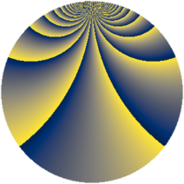# Properties

 Label 2240.2.egLevel $2240$ Weight $2$ Character orbit 2240.eg Rep. character $\chi_{2240}(267,\cdot)$ Character field $\Q(\zeta_{16})$ Dimension $2304$ Sturm bound $768$

# Related objects

## Defining parameters

 Level: $$N$$ $$=$$ $$2240 = 2^{6} \cdot 5 \cdot 7$$ Weight: $$k$$ $$=$$ $$2$$ Character orbit: $$[\chi]$$ $$=$$ 2240.eg (of order $$16$$ and degree $$8$$) Character conductor: $$\operatorname{cond}(\chi)$$ $$=$$ $$320$$ Character field: $$\Q(\zeta_{16})$$ Sturm bound: $$768$$

## Dimensions

The following table gives the dimensions of various subspaces of $$M_{2}(2240, [\chi])$$.

Total New Old
Modular forms 3104 2304 800
Cusp forms 3040 2304 736
Eisenstein series 64 0 64

## Trace form

 $$2304q + O(q^{10})$$ $$2304q + 64q^{12} - 64q^{22} - 32q^{24} + 80q^{30} - 112q^{38} + 80q^{40} + 208q^{48} + 208q^{50} + 64q^{51} - 96q^{56} - 112q^{60} + 112q^{68} - 128q^{69} - 240q^{72} - 128q^{76} + 64q^{79} + 160q^{82} - 224q^{87} + 304q^{92} - 256q^{99} + O(q^{100})$$

## Decomposition of $$S_{2}^{\mathrm{new}}(2240, [\chi])$$ into newform subspaces

The newforms in this space have not yet been added to the LMFDB.

## Decomposition of $$S_{2}^{\mathrm{old}}(2240, [\chi])$$ into lower level spaces

$$S_{2}^{\mathrm{old}}(2240, [\chi]) \cong$$ $$S_{2}^{\mathrm{new}}(320, [\chi])$$$$^{\oplus 2}$$